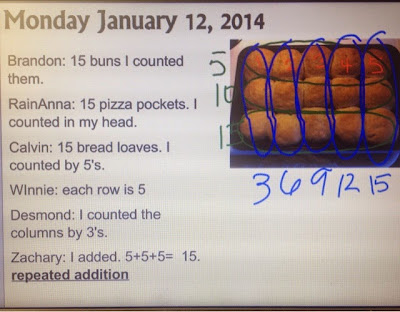## Tuesday, January 13, 2015

### Measurement

Our current focus in math is on Measurement. We have already explored measuring in centimeters (CM) and meters (M). Students are measuring different objects and deciding what the best unit for measurement should be.

We are now moving to recall how to estimate, measure and record the perimeter of a shape or an object. Students are playing games and practicing how to measure the perimeter of various shapes. Ask your child what they have learned about measuring using a CM ruler so far.To begin our math lessons this week, we looked at some images and wondered what math we see. Students are discovering so much math in simple images. It is pretty amazing to see what our class has discovered so far. Have a look at some of our math discussions!